# Venn Diagram Calculator

Created by Davide Borchia
Reviewed by Rijk de Wet
Last updated: Apr 03, 2023

The Venn diagram calculator is a comprehensive tool that lets you compute all the relevant quantities in two and three-set Venn diagrams. Even though we learn to deal with sets from a young age, set theory is a complex branch of math — keep reading this article to learn:

• What Venn diagrams are;
• How to calculate Venn diagrams, with calculations for 2-circle Venn diagram and 3-circles (shading calculator and numerical calculations); and
• How hard is it to calculate a 4-circle Venn diagram?

## What are Venn diagrams? Introduction to set theory

A Venn diagram is a representation technique in set theory that allows you to visualize sets as closed curves, by convention either circles or ellipses. A set $A$ is a collection of elements: we are most interested in the cardinality (i.e., the number of elements or the size) of the sets, which is denoted as $|A|$. When more than one set appears in a diagram, we can start defining relationships between them: there's the union, the intersection, and a whole list of set operations that only marginally resembles the ones we perform on numbers.

Let's jump to these operations and learn how to calculate a Venn diagram set's cardinality in the most basic example: two sets.

## Venn diagram calculations for a 2 circle diagram

The first non-trivial Venn diagram involves two sets. You can see its most known representation in the picture below.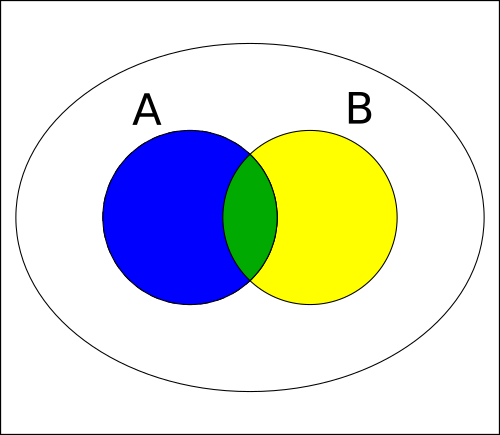In this simple diagram, we can start identifying the various sets of interests:

• The sets $A$ and $B$.

• The intersection between $A$ and $B$: $A\cap B$. This subset contains all the elements that belong both to $A$ and to $B$.

• The union of $A$ and $B$: $A \cup B$. The elements in this set belong to $A$ or $B$.

• The set differences: in this case we deal with elements that belong exclusively to $A$ (the subset $A\text{\textbackslash}B$) or that belong exclusively to $B$ (the subset $B\text{\textbackslash}A$).

• The symmetric difference of the sets $A$ and $B$. This set corresponds to the logical operation XOR, or "exclusive or" (visit the XOR calculator if you need a quick explanation of this operator). The set $A\Delta B$ contains all the elements that belong to $A$ or to $B$, but not to both.

🙋 For a quick refresh on unions and intersections, visit our union and intersection calculator.

#### Calculations in a 2-circle Venn diagram

Calculating the cardinality of the sets in a 2-circle Venn diagram is never too complex. However, the formulas of choice will depend greatly on the information you know about the problem. First and foremost, it lets you calculate the intersection from the union and vice-versa. In mathematical terms, this is the inclusion-exclusion principle:

$\footnotesize |A\cup B| = |A| + |B| - |A\cap B|$

Notice how we used the cardinality of the sets. The intersection's cardinality appears with a negative sign because we considered the intersection's elements twice while summing the cardinalities of $A$ and $B$.

Once we know either the union or the intersection, we can calculate the set differences:

$\footnotesize\begin{split} |A\text{\textbackslash}B| &= |A| - |A\cap B|\\[.5em] |B\text{\textbackslash}A|& = |B| - |A\cap B| \end{split}$

The symmetric difference is the sum of these two results, but we can also write it neatly:

$\footnotesize|A\Delta B| = |A\cup B| - |A\cap B|$

## 3-set Venn diagram calculations

Let's increase the number of circles to 3. As you can see from the image below, the number of sets increases markedly.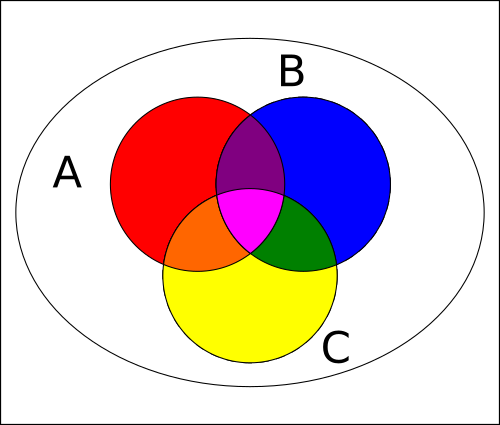Now, for every pair of sets, we can define an intersection ($A\cap B$, $A\cap C$, $B\cap C$), and on top of them, an intersection between all their sets ($A\cap B\cap C$). A similar approach applies to the union too.

To compute the union and intersection of the sets, we can use once again the inclusion-exclusion principle, but this time we will see it in a more complex form:

$\footnotesize \begin{split} |A\cup B\cup C| &= |A| + |B| + |C|\\[.5em] &\!\!\!\!\!\!\!\!\!\!\!\!\!\!\!- |A\cap B| - |A\cap C| -|B\cap C| \\[.5em] &\!\!\!\!\!\!\!\!\!\!\!\!\!\!\!+ |A\cap B\cap C| \end{split}$

The sets in the second rows are the cardinalities of the intersections between each pair of sets: to calculate the union of $A$, $B$, and $C$, you need to know those values, even though they are not of immediate importance.

In a 3-set Venn diagram, we calculate the difference between sets by knowing the value of the unions between each pair of sets or by carefully erasing the intersections from a set. In mathematical terms, this corresponds to these two formulas:

$\footnotesize \!|A\text{\textbackslash}(B\cup C)|\! =\! |A\cup B\cup C| \!-\! |B\cup C|$
$\footnotesize \begin{split} \!|A\text{\textbackslash}(B\cup C)|\! &=\! |A| \!-\! |A\cap B|\!-\! |A\cap C|\\[.5em] & \!+\! |A\cap B\cap C| \end{split}$

The first one uses unions of sets. The second one, which uses their intersections, requires a subtraction that erased the same set twice: in this case, we need to sum the 3-set intersection, too.

The last quantity we can calculate is the symmetric difference. In this instance, we will use a property of this operation: associativity:

$\footnotesize A \Delta B\Delta C =( A\Delta B)\Delta C$

This means we can calculate it first for two sets (something we know how to do), then consider the symmetric difference between the result and the third set. The result is an interesting figure: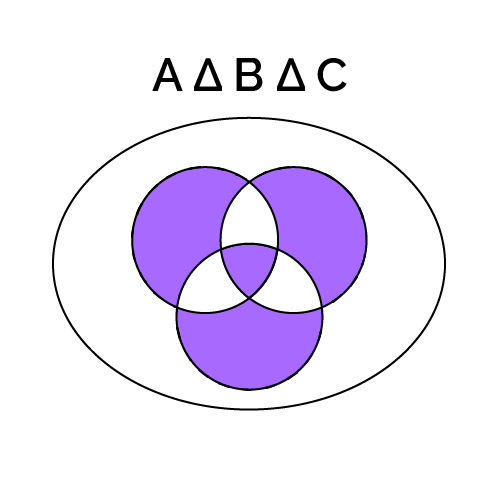In this image, you can also see how the symmetric difference corresponds to the modulo 2 addition of the sets. Discover how to perform this operation at Omni's modulo calculator.

## Increased complexity: some calculations for the 4 circle Venn diagram

Add one set. Now we are dealing with the four-set Venn diagram. To build a representation of it, we need to move away from circles: ellipses help create a diagram where each set appears once and only once.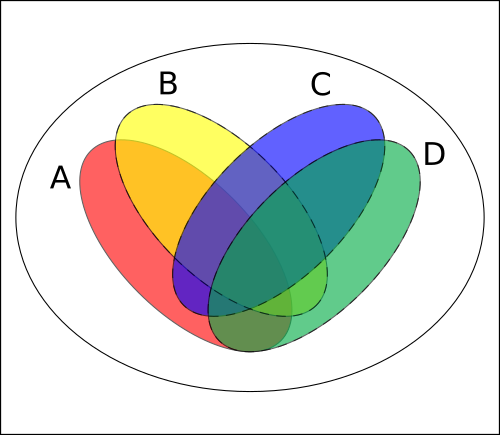Here we can identify 15 different sets: four set differences, six double intersections, four triple intersections, and one quadruple intersection. The complexity grows quickly enough that calculations can be performed only knowing a vast amount of data (14 out of the 15 sets, for example). In mathematical terms, we say that the number of sets grows with the binomial coefficient.

The situation is even more dramatic for a 5-set Venn diagram. In this case, we will only leave a representation of the symmetric difference here. Each of the purple-colored elements in this set must be computed individually. There are 16 subsets that together contribute to form this interesting, flower-like shape.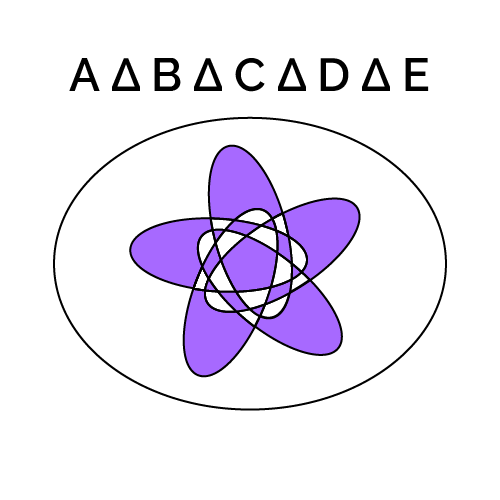## Enter the universe

For each of the quantities we listed above, we can define a complement. The complement of a set, in set theory, is the set that adds a "not" to the definition of the set it refers to. The complement of the intersection of $A$ and $B$ is the set that does not contain any element shared by both $A$ and $B$. To define a complement, we need to define what's outside of our set.

In mathematical language, the set of everything we are not interested in is the universe, and we can represent it with a large set that encompasses our Venn diagram. In terms of notations, wrap your set in brackets (if needed) and add an apostrophe at the end. In the picture below, you can see the complement of the symmetric difference of three setsand the corresponding notation: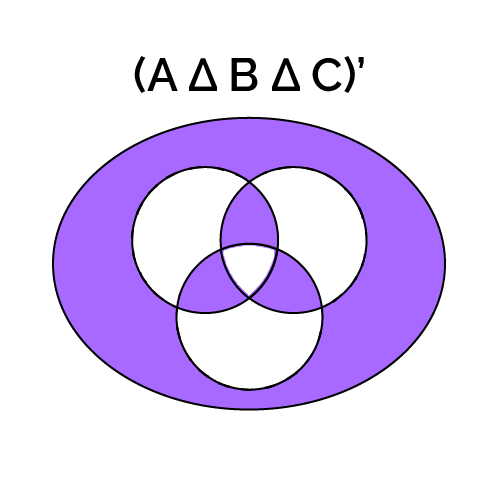## How to use Omni's Venn diagram calculator?

Omni's Venn diagram calculator allows you to calculate all the sets and subsets, and complements of 2-set and 3-set Venn diagrams.

The first thing you have to do is choose how many sets appear in your Venn diagram. Select the proper number in the topmost variable. Then, fill the fields with known values. If we can run some calculations on your provided data, we will do so in the blink of an eye!

🙋 If you are confused by the notation, worry not: click on the name of the set/subset you are interested in, and graphics explaining which quantity corresponds to that label will appear in a new small window!

Maybe, reading this article, you noticed some similarities with another field of science: probability. Visit our probability calculator for 3 events to discover where set theory and probability theory meet.

## FAQ

### What is the inclusion-exclusion principle?

The inclusion-exclusion principle is a fundament of set theory, a formula that allows you to calculate the union or intersection of any number of sets. To calculate the union, follow these easy steps:

1. Sum the cardinalities of the individual sets.
2. Subtract the cardinality of the intersections of each pair of sets.
3. Sum the cardinality of each intersection of three sets.

This alternate fashion of sum and difference repeats until you end up with possible intersections. This operation results in the cardinality of the union of the sets.

### How do I calculate the intersection between three sets?

To calculate the intersection between three sets, A, B, and C, you can use the inclusion-exclusion principle. We assume you will know all the quantities mentioned.

1. Find the cardinality of the union of all three sets (|A ∪ B ∪ C|).
2. Subtract the cardinality of every single set(|A|, |B|, and |C|).
3. Add the cardinality of the intersection of each pair of set (|A ∩ B|, |A ∩ C|, and |B ∩ C|).

The result will be the cardinality of the intersection |A ∩ B ∩ C|.

### What is the union if |A| = 10, |B| = 12, and |A ∩ B| = 4?

The union of A and B, with |A| = 10 and |B| = 12 is |A ∪ B| = 18. To find this result, we use the inclusion-exclusion principle:

1. Calculate the sum of the cardinalities |A| and |B|: |A| + |B| = 10 + 12 = 22.
2. Subtract the cardinality of their intersection: |A| + |B| - |A ∩ B| = 22 - 4 = 18.

That's it. We subtracted the intersection because, by summing |A| and |B|, we inadvertently counted twice the elements shared by the two sets.

### What is the symmetric difference of two sets?

The symmetric difference of two sets, A and B, is the set that contains all the elements belonging exclusively to either A or B. The corresponding logical operation is the exclusive or: when both sets exist in a given portion of the diagram, we disregard it. With higher numbers of sets in your diagram, use sum modulo 2 of the overlaps of the sets. If the overlaps are even, exclude the subset. If the overlaps are odd, include the subset.

Davide Borchia
Number of sets
2
|U|
|A|
|B|
|A\B|
|B\A|
|A∪B|
|A∩B|
|AΔB|
Complements
|A'|
|B'|
|(A\B)'|
|(B\A)'|
|(A∪B)'|
|(A∩B)'|
|(AΔB)'|
People also viewed…

### Accuracy

The accuracy calculator is a simple tool for calculating accuracy using three simple methods.

### Body fat

Use the body fat calculator to estimate what percentage of your body weight comprises of body fat.

### Podcasts

Do you feel like you could be doing something more productive or educational while on a bus? Or while cleaning the house? Well, why don't you dive into the rich world of podcasts! With this podcast calculator, we'll work out just how many great interviews or fascinating stories you can go through by reclaiming your 'dead time'!

### Simpson’s diversity index

Use Omni's Simpson's diversity index calculator to measure the species diversity in a community.